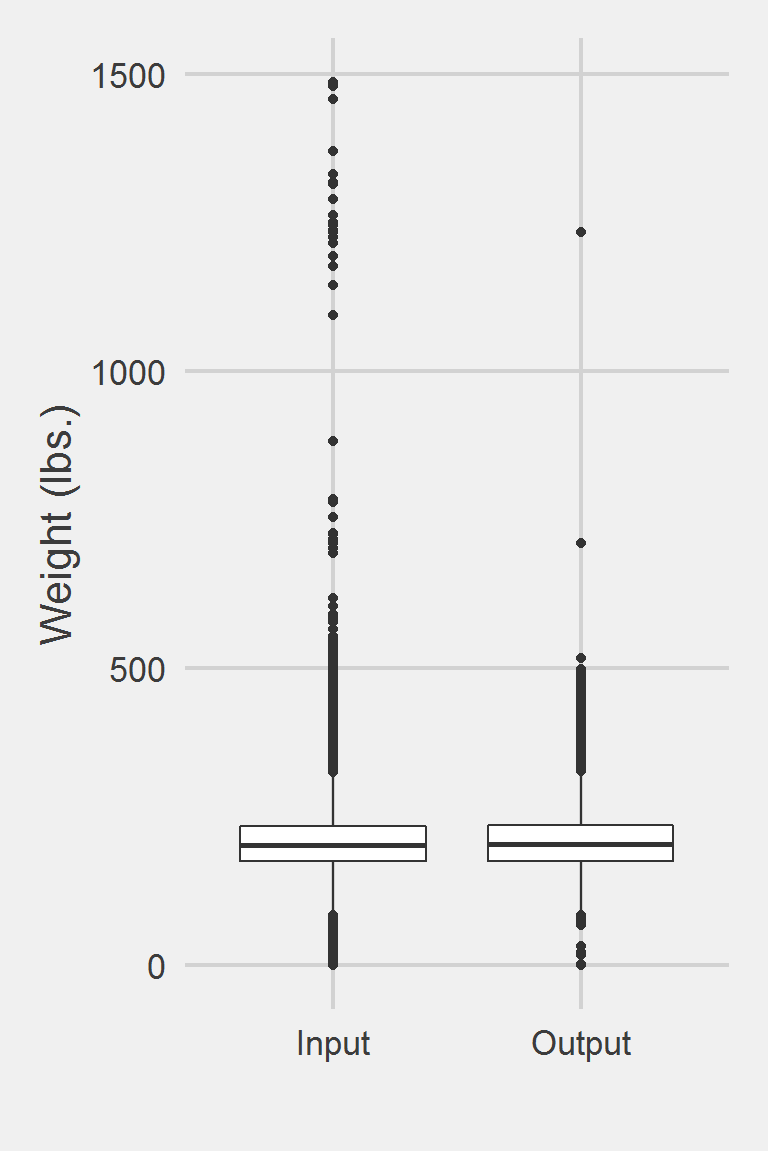## Kazerooni 2016

### Excerpt from published methods

Patients were excluded from this study if they were missing weight values from any of the three measuring points of the study (pre-, mid-, and post-weight) … Pre-weight was defined as the most recent weight within 30 days before starting topiramate therapy. Mid-study period was defined as the earliest weight taken between 3 and 6 months post initiation of therapy. Post-study period was defined as the earliest weight taken between 6 and 12 months post initiation of therapy.

### Translation in pseudocode

DEFINE time t_ij for person i IN 1:I, j weights In 1:J {pre-trt, mid-trt, post-trt}
FOR i IN 1:I
FOR j IN 1:J
weight_i1 := weight @ t_0 (treatment start) - 30 days
weight_i2 := {t_0 + 90 days <= weight <= t_0 + 180 days}
weight_i3 := {t_0 + 180 days < weight <= t_0 + 365 days}
END FOR
IF (weight_ij IS NULL) (weight measure missing at jth time point)
EXCLUDE person i
END FOR

### Algorithm in R Code

#' @title Kazerooni et al. 2016 Weight Cleaning Algorithm
#' @param DF object of class data.frame, containing id and measures
#' @param id string corresponding to the name of the column of patient identifiers in DF
#' @param measures string corresponding to the name of the column of measures in DF, e.g., numeric weight data if using to clean weight data.
#' @param tmeasures string corresponding to the name of the column of measure dates and/or times in DF
#' @param startPoint string corresponding to the name of the column in DF holding the time at which subsequent measurement dates will be assessed, should be the same for each person. Eg., if t = 0 (t) corresponds to an index visit held by the variable 'VisitDate', then startPoint should be set to 'VisitDate'
#' @param t numeric vector of time points to collect measurements, eg. c(0, 182.5, 365) for measure collection at t = 0, t = 180 (6 months from t = 0), and t = 365 (1 year from t = 0). Default is c(0, 182.5, 365) according to Kazerooni et al. 2016
#' @param windows numeric list of two vectors of measurement collection windows to use around each time point in t. E.g. Kazerooni et al. 2016 use c(30, 0, 0) for the lower bound and c(0, 0, 185) for the upper bound at t of c(0, 90, 180), implying that the closest measurement to t (=0) will be within the window [-30, 0], then the closest to t (=90) will be within [90, 180], t (=180) within (180, 365]
Kazerooni2016.f <- function(DF,
id,
measures,
tmeasures,
startPoint,
t = c(0, 90, 180),
windows = list(LB = c(30, 0, 0),
UB = c(0, 90, 185))) {

if (!require(dplyr)) install.packages("dplyr")
if (!require(rlang)) install.packages("rlang")

tryCatch(
if (class(DF[[tmeasures]]) != class(DF[[startPoint]])) {
stop(
print(
paste0("date type of tmeasures (",
class(DF[[tmeasures]]),
") != date type of startPoint (",
class(DF[[startPoint]]),
")"
)
)
)
}
)

tryCatch(
if (class(t) != "numeric") {
stop(
print("t parameter must be a numeric vector")
)
}
)

tryCatch(
if (!is.list(windows)) {
stop(
print("windows must be placed into a list object")
)
}
)

# compute difference in time between t0 and all t_j
id         <- rlang::sym(id)
tmeasures  <- rlang::sym(tmeasures)
startPoint <- rlang::sym(startPoint)

DF <- DF %>%
mutate(
time = as.numeric(
difftime(
!!tmeasures, !!startPoint,
tz = "utc", units = "days"
)
)
)

# loop through each time point in t, place into list
meas_tn <- vector("list", length(t)) # set empty list
for (i in 1:length(t)) {
meas_tn[[i]] <- DF %>%
filter(time >= t[i] - windows$LB[i] & time <= t[i] + windows$UB[i]) %>%
group_by(!!id) %>%
arrange(abs(time - t[i])) %>%
slice(1)
}

# count number of time points available for each subject i
do.call(rbind, meas_tn) %>%
arrange(!!id, !!tmeasures) %>%
group_by(!!id) %>%
filter(max(row_number()) >= 3) %>% # must have all 3 time points
ungroup()
}

### Algorithm in SAS Code

#TODO

### Example in R

Kazerooni2016.df <- Kazerooni2016.f(DF,
id = "PatientICN",
measures = "Weight",
tmeasures = "WeightDateTime",
startPoint = "VisitDateTime")

Distribution of Weight Measurements between Raw and Algorithm-Processed Values


Descriptive statistics by group
group: Input
vars       n   mean   sd median trimmed   mad min    max  range skew
X1    1 1175995 207.82 48.6  202.3  204.62 44.18   0 1486.2 1486.2 0.98
kurtosis   se
X1      5.6 0.04
------------------------------------------------------------
group: Output
vars     n mean    sd median trimmed   mad min    max  range skew kurtosis
X1    1 71961  209 47.95    204  206.02 44.33   0 1233.7 1233.7 0.88     4.27
se
X1 0.18

We won’t show a vignette for Kazerooni & Lim 2016, as it just excludes people, retaining only those with a certain number of measurements.

Left boxplot is raw data from 2016, PCP visit subjects while the right boxplot describes the output from running Kazerooni2016.f()